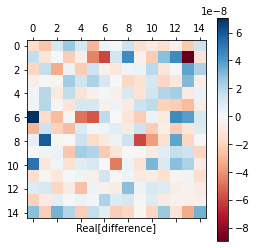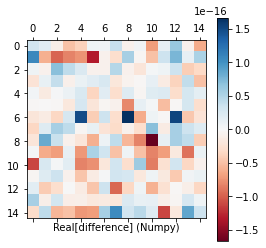# Incorrect SVD using `jax.linalg.svd` - Python jax

I have a code that uses singular value decomposition (SVD) to obtain an operator and then compute the Jacobian for it using `jacfwd`. But I was getting NaNs so I tried to test the implementation of SVD in Jax for complex matrices. The following snippet shows one instance where the Jax implementation of SVD fails with errors of order 1e-7. With the suggestion by @hawkinsp I tried using 64-bit precision and still see an error. This error somehow depends on the singular values and it is possible to get larger errors in some cases.

``````import numpy as np
from scipy.stats import unitary_group

from jax.config import config
from jax import numpy as jnp
import jax

print("Jax version", jax.__version__)
config.update("jax_enable_x64", True)

np.random.seed(42)

N = 15

# Construct an operator using two random Unitaries
U = unitary_group.rvs(N)
V = unitary_group.rvs(N)
S = np.zeros((N, N))
np.fill_diagonal(S, np.random.uniform(0.5, 1., size = (N, N)))

op = np.dot(U*S, jnp.conjugate(V).T).astype(jnp.complex128)

u, s, v = jnp.linalg.svd(op)
assert(np.allclose(op, jnp.dot(u*s, v)))
``````

which results in

``````Jax version 0.1.70
---------------------------------------------------------------------------
AssertionError                            Traceback (most recent call last)
<ipython-input-79-babe60e251fc> in <module>
25
26 u, s, v = jnp.linalg.svd(op)
---> 27 assert(np.allclose(op, jnp.dot(u*s, v)))

AssertionError:
``````

Numpy however gives the correct decomposition with

``````u, s, v = np.linalg.svd(op)
np.allclose(op, np.dot(u*s, v))
``````

If I compare the error in the reconstructed matrix after SVD, it is of the order 1e-7 using Jax:Numpy however gives a much better result with errors of the order 1e-16:Is it possible to test the svd function better (or with lower tolerances) since right now the tests are with tol=1e-4 and rtol=1e-4 in https://github.com/google/jax/blob/master/tests/linalg_test.py#L584

@hawkinsp makes a comment that the tests are very loose. I am wondering if this is leading to issues in my code since I have a forward function that takes SVD and then removes negative singular values and computes a Jacobian for the resulting matrix. I get NaNs for such a computation.quantshah

Your test passes for me exactly as written at head, on CPU.

Can you please (a) use an up to date JAX (0.2.9 is current), (b) describe what hardware platform you are using, and (c) give a test case that fails?

An error of `1e-7` sounds an awful lot like you are still using single precision. The `jax.config.update` call must be one of the first things you do when calling JAX; if you are using something like using Colab or Jupyter, make sure to restart the kernel and try again.

1
Answered Feb 19 '21 at 16:06hawkinsp

Thanks @hawkinsp. You are right, I am on an older version and should have restarted the kernel. I tried it now on Colab and it works if my matrix is of type `complex128`!

However, if I am using `complex64`, I get errors with the following code on Colab:

``````import numpy as np
from scipy.stats import unitary_group

from jax.config import config
from jax import numpy as jnp
import jax

print("Jax version", jax.__version__)
config.update("jax_enable_x64", True)

np.random.seed(42)

N = 53

# Construct an operator using two random Unitaries

U = unitary_group.rvs(N)
V = unitary_group.rvs(N)
S = np.zeros((N, N))
np.fill_diagonal(S, np.random.uniform(-10, 10., size = (N, N)))

op = np.dot(U*S, jnp.conjugate(V).T).astype(jnp.complex64)

u, s, v = jnp.linalg.svd(op)
assert(np.allclose(op, jnp.dot(u*s, v)))
``````

I guess it is just due to some issue with precision that I am not so familiar with and should understand more.

1
Answered Feb 19 '21 at 16:18quantshah

My guess is that NumPy may be computing with 64-bit precision internally.

I computed the norm of the difference between the two matrices and found it was around 4e-6. I think that's all we can really expect for single-precision computation. If you want more precision, use double precision!

I hope that helps!

1
Answered Feb 19 '21 at 16:28hawkinsp

Thank you so much. It helps a lot and I will read up more about the issue now.

1
Answered Feb 19 '21 at 16:50quantshah